Request a call back

<div>how to balance chemical equations easily</div> <div>&nbsp;</div> <div>&nbsp;</div>
Asked by Suvrajyoti | 08 Aug, 2016, 12:01: AMExpert Answer

Steps involved in Balancing a Chemical Equation

Consider the chemical reaction between magnesium and oxygen to understand the steps involved in balancing a chemical equation.

Step 1

Let us first write the word equation for this reaction.

Magnesium + Oxygen → Magnesium oxide

Step 2

Write the chemical equation for the reaction between magnesium and oxygen.

Mg + O2  → MgO

Step 3

Count the number of times an element is occurring on both LHS and RHS in this equation.

Mg  +  O2  →  MgO

 Component Reactant Product Magnesium 1 1 Oxygen 2 1

This is an unbalanced equation.

Step 4

• To balance a chemical equation, first draw boxes around each formula. Do not change anything inside the boxes while balancing the equation.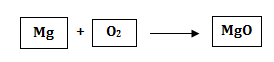• Choose a reactant or a product that has the maximum number of atoms in it. In that compound, select the element which has the maximum number of atoms. In this equation we shall select MgO i.e. magnesium oxide and the element oxygen in it.
• To balance the oxygen atoms, let us multiply magnesium oxide molecule by 2 on the right hand side.

The equation can now be expressed as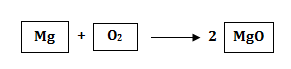Component Reactant Product Magnesium 1 1 X 2 = 2 Oxygen 2 1 X 2 = 2

Step 5

There are two oxygen atoms on either side of the equation, but one magnesium atom on the reactant's side and two on the product's side. Therefore, multiply the magnesium atom by 2 on the left hand side.

 Component Reactant Product Magnesium 1 X 2 = 2 2 Oxygen 2 2

The balanced equation is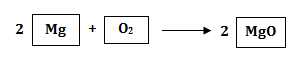The number of atoms of each element of the reactants = The number of atoms of each element of the products

Step 6

Writing Specific conditions on the Arrow

The reaction is carried out in the presence of ‘heat’. On heating, magnesium combines with the oxygen present in the air to form magnesium oxide.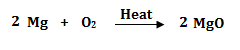Step 7

Writing symbols of the Physical state

• To make a chemical equation more informative, the physical states of the reactants and products are mentioned along with their chemical formulae.
• The gaseous, liquid, aqueous and solid states of the reactants and products are represented by the notations (g), (l), (aq) and (s) respectively.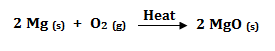Using these steps, you can balance any chemical equation.

Answered by Vaibhav Chavan | 08 Aug, 2016, 10:54: AM